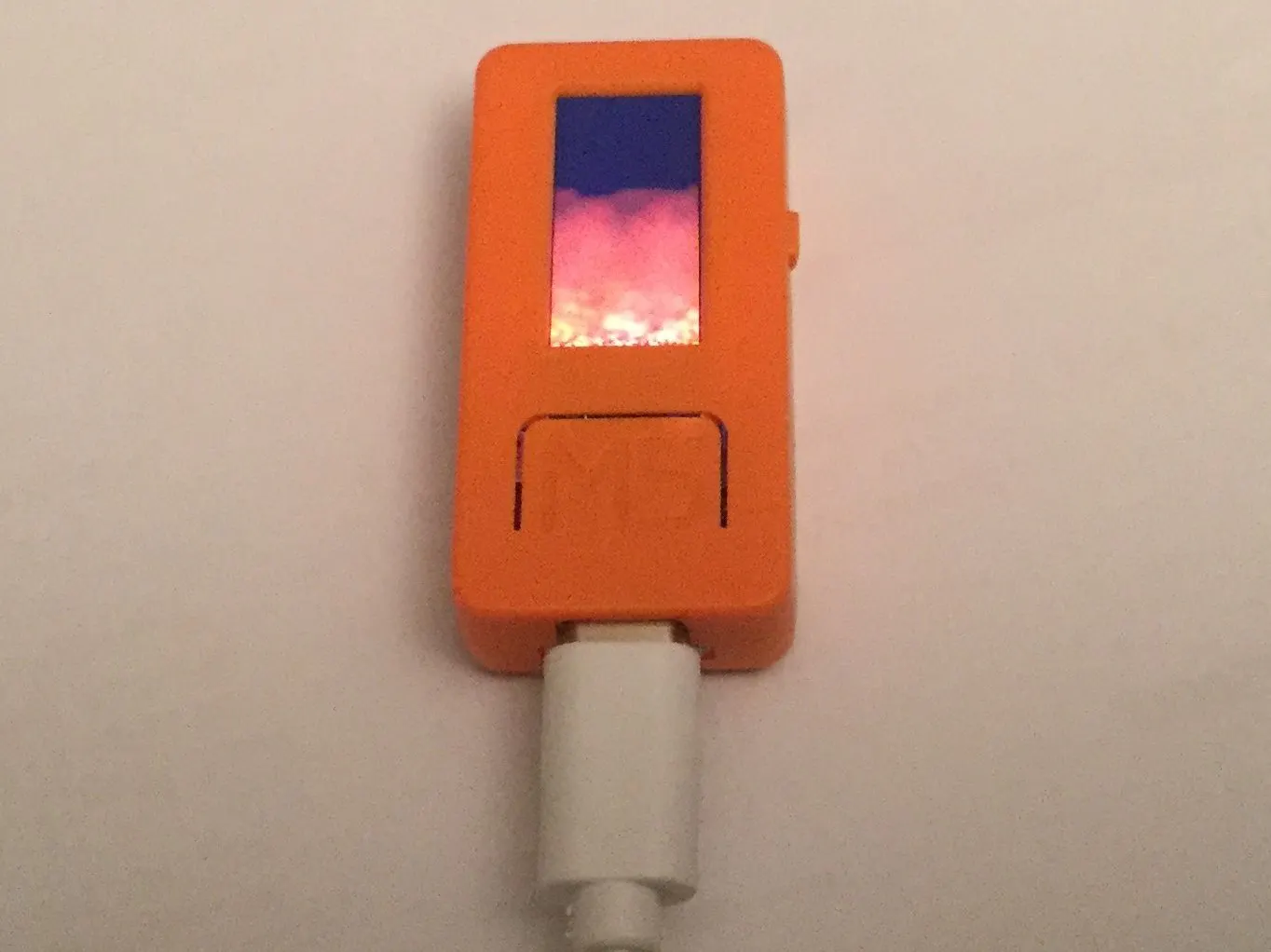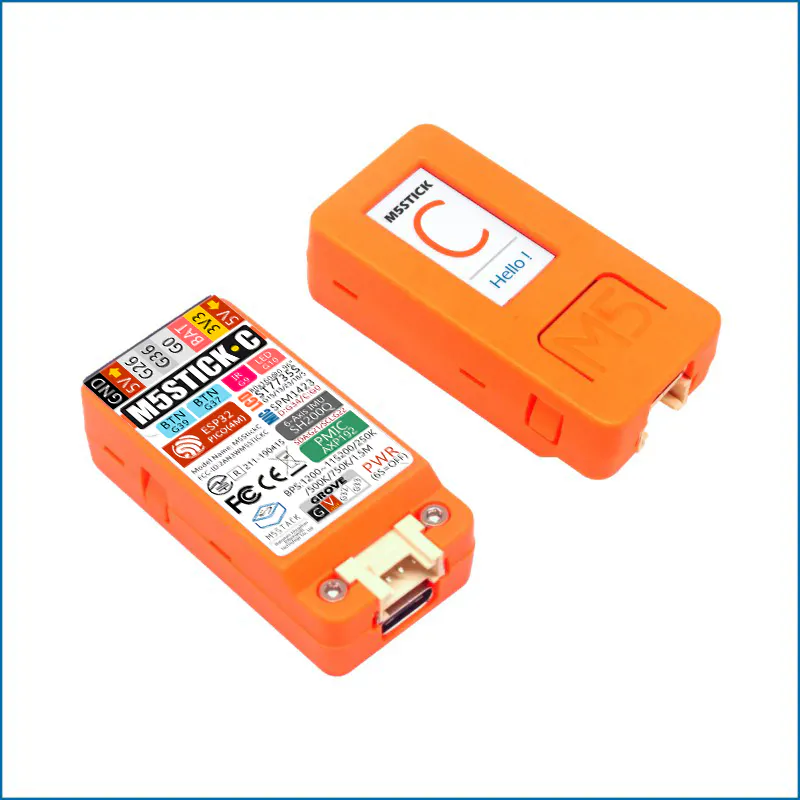# M5StickC Flame Demo

Oldschool fire demo

IntermediateWork in progress1,015## Things used in this project

### Hardware componentsM5Stack M5StickC ESP32-PICO Mini IoT Development Board
×1

### Software apps and online servicesArduino IDE

## Code

### M5StickC_Flame

Arduino
M5StickC Arduino IDE code
```// Simple M5StickC oldschool flame effect
// All credit to Lode Vandevenne https://lodev.org/ whose code I have adapted for the M5StickC

#include <M5StickC.h>

const int screenWidth = 80;
const int screenHeight = 160;
int colour; // Array to hold the colour palette
int fire[screenHeight][screenWidth]; //Array to hold the screen buffer

void setup() {
// put your setup code here, to run once:
M5.begin();

// This for loop creates a black -> red then red -> yellow colour palette
for (int i = 0; i < 128; i++) {
if (i < 64) {
colour[i] = (int(i/2) * 0x800);
}
else {
colour[i] = 0xf800 + ((i - 64) * 0x20);
}
}

// Clear the screen buffer

for (int y = 0; y < screenHeight; y++) {
for (int x = 0; x < screenWidth; x++) {
fire[y][x] = 0;
}
}
}

void loop() {
// put your main code here, to run repeatedly:

// Randomise the bottom line of the screen buffer

for (int x = 0; x < screenWidth; x++) {
fire[screenHeight - 1][x] = (64 + random(64));
}

// Calculate the flame algorithm from the top down

for (int y =0; y < (screenHeight - 1); y++) {
for (int x = 0; x < screenWidth; x++) {
fire[y][x] =
((fire[(y + 1) % screenHeight][(x - 1) % screenWidth]
+ fire[(y + 1) % screenHeight][(x) % screenWidth]
+ fire[(y + 1) % screenHeight][(x + 1) % screenWidth]
+ fire[(y + 2) % screenHeight][(x) % screenWidth])
* 64) / 257; // play with these values for different effects, second number must be just over 4 x the first
}
}

// Update the screen

for (int y =0; y < screenHeight; y++) {
for (int x = 0; x < screenWidth; x++) {
M5.Lcd.drawPixel(x, y, colour[(fire[y][x])]);
}
}
}
```

### M5Stack Flame Demo

Arduino
Changes to the M5StickC code to run on the M5Stack
```// Simple M5Stack oldschool flame effect
// All credit to Lode Vandevenne https://lodev.org/ whose code I have adapted for the M5Stack

#include <M5Stack.h>
// Only using every other pixel due to speed and memory use
const int screenWidth = 160;
const int screenHeight = 120;
int colour; // Array to hold the colour palette
int fire[screenHeight][screenWidth]; //Array to hold the screen buffer

void setup() {
// put your setup code here, to run once:
M5.begin();

// This for loop creates a black -> red then red -> yellow colour palette
for (int i = 0; i < 96; i++) {
if (i < 32) {
colour[i] = (i * 0x800);
}
else {
colour[i] = 0xf800 + ((i - 32) * 0x20);
}
}

// Clear the screen buffer

for (int y = 0; y < screenHeight; y++) {
for (int x = 0; x < screenWidth; x++) {
fire[y][x] = 0;
}
}
}

void loop() {
// put your main code here, to run repeatedly:

// Randomise the bottom line of the screen buffer

for (int x = 0; x < screenWidth; x++) {
fire[screenHeight - 1][x] = (32 + random(64));
}

// Calculate the flame algorithm from the top down

for (int y =0; y < (screenHeight - 1); y++) {
for (int x = 0; x < screenWidth; x++) {
fire[y][x] =
((fire[(y + 1) % screenHeight][(x - 1) % screenWidth]
+ fire[(y + 1) % screenHeight][(x) % screenWidth]
+ fire[(y + 1) % screenHeight][(x + 1) % screenWidth]
+ fire[(y + 2) % screenHeight][(x) % screenWidth])
* 64) / 257 ; // play with these values for different effects, second number must be just over 4 x the first
}
}

// Update the screen

for (int y =0; y < screenHeight; y++) {
for (int x = 0; x < screenWidth; x++) {
M5.Lcd.drawPixel(2*x, 2*y, colour[(fire[y][x])]);
}
}
}
```

## Credits

### MajorSnags

1 project • 0 followers